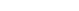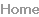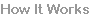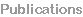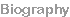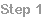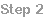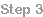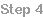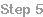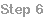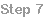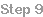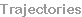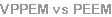Trajectories
Our basic explanation is split into several stepsTrajectory calculations using the vector potential
Usually electron trajectory calculations use the magnetic field strength and the Lorenz force. Here we
calculate the vector potential field directly,and use force equations derived from the change in momentum.
In the VPPEM the momentum from the vector potential is converted
into the off-axis momentum of an angular image by suddenly
terminating the field.

To understand this action in detail we have created a computer
program to simulate an electron trajectory where the Lorenz force has
been rewritten using the forces due to changes in the vector potential.
The vector potential is given by equation 1: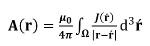(1)
Where A(r) is the vector potential at position r, J(r ́ ) is the current
density at position r ́, and Ω is the volume of the current density.
Integrating Equation 1 around a circular loop of current gives a vector
potential field that is zero on the axis, and is a maximum at the radius.

The direction of the momentum is around the axis in the direction of
the current. The curl (∇×) of this field distribution is a constant. From
Maxwell’s equations the magnetic field is: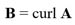(2)
The canonical momentum of a charged particle in a magnetic field is: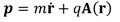(3)
Where p is the momentum, m is the electron mass, and q is the
electron charge.

Using standard analytical mechanics we write the Lagrangian for an
electron in a vector potential field: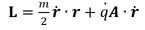(4)
After using Lagrange’s equations: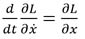(5)We obtain the resolved force equations:
(6)
(7)
(8)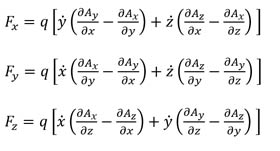The force equations are used to track trajectories in a simple model system.
Figure 1 shows a model system with two current loops.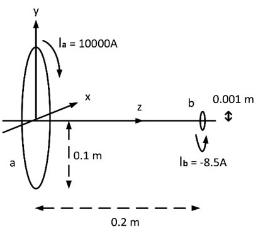Figure 1. Two current loop model for electron trajectory simulations using the
vector potential. The current in loop b is opposite to the current in loop a, and
reduces the total field at the center of loop b to zero.
Page 1
We have arranged the respective currents in the current loops to produce a
zero field at the center of the second loop. After passing the second loop the
field is numerically set to zero to simulate the electron passing through a
ferromagnetic shield.
All terms in Az are zero in equations 6-8 because there is no resultant
vector potential out of the plane of the current loops.

For any position in the electron trajectory Ax and Ay, and their
differentials are numerically calculated by integrating equation 1 around
the current loops.

The initial field at the center of loop a along the z axis, Bz, is calculated
from the curl of the vector potential, or simply:(9)
From the dimensions and current shown in Figure 1 the initial
Bz = 0.06283 Tesla, in agreement with the standard calculation.
The shape of the axial B field with z is shown in Figure 2.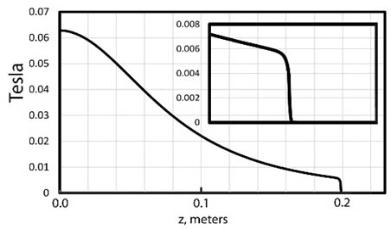Figure 2 Axial magnetic field Bz of the model in Figure 1. Inset is a detail that
shows the ‘knee’ of the magnetic field.
Starting at z=0, x=0, and y=10 microns the simulated electron trajectory
moves smoothly along a field line with its distance from the axis in y given
approximately by: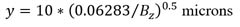(10)
With higher energy electrons there is a significant deviation from this
relationship due to non-adiabatic behavior. This is seen in the additional
deflection in the x direction.

Figure 3 shows the results of a simulation of a 2.0 eV electron leaving the
central field parallel to the z axis with an initial y offset of 10 microns.
Figure 3 shows that the final angle of the trajectory in the y direction is
largely determined by the curve in the trajectory following the field line.

In contrast, the angle of the trajectory in the x direction is directly due to the
force created by the termination of the field.

The combination of the two deflections causes a rotation of the trajectory
out of the y plane.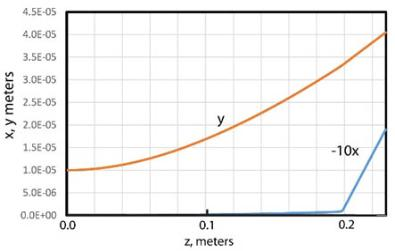Figure 3 A simulated 2.0 eV electron trajectory resolved on the x and y axis for
the model shown in Figure 1.
Page 2The angle of rotation is a constant of the model system, and does not
depend on the starting position in x and y.

The resultant angular images have two angular properties, the first in the
direction of the magnetic vector potential (φ the transverse rotational
direction, the x direction), and the second in the response to the magnetic
field lines diverging from the axis at the field termination (θ the off-axis
inclination, the y direction)
It can also be seen that the apparent position of the source of the angled
trajectory is at a significantly different position along the z axis for the x and
y directions. This shift in position makes a true focus of a bundle of
Figure 4 shows the forces in Fx and Fy in the area of the knee in the
magnetic field. As the electron trajectory approaches the ‘knee’ in the
magnetic field due to the opposing small loop field, it sees a rapid
change in the magnetic field going to zero at the center of the second
loop, and the trajectory is no longer adiabatic.

The force due to the change in the vector potential in the x direction
increases more than the force in the y direction, and the electron is
deflected away from the axis.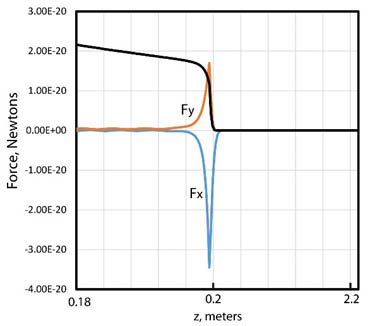Figure 4 Resolved forces on an electron at the termination of the magnetic
field: x (blue line), y (red line). The forces rapidly increase as the electron is
driven away from the magnetic field line, and then decay as the electron leaves
the field. The actual position of the magnetic field knee is shown
superimposed as the black line.